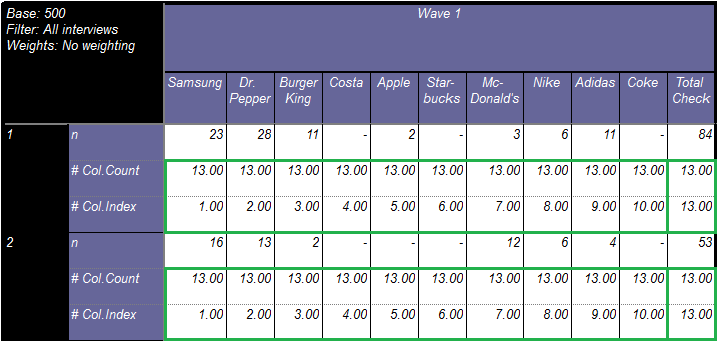Scroll

# Script Calculations for Tables Repeated by Edge

Follow
 Summary This article shows the use of calculation arithmetic with scripts to create a simple bespoke calculation in our table of only rows and columns. It then goes on to demonstrate how the script can be amended to work with or without questions in the edge. Applies to Askia Analyse. Written for Researchers, Developers, Data Processors. Keywords Table arithmetic, Forloops, Coordinates, data, Calculation arithmetic, Bespoke calculation, Repeated table, Edges, Filtered.

The .qes file and portfolio containing the example discussed is attached: Table Arithmetic Examples 9.rar.

This article expands upon the introduction to Table Arithmetic given in our Analyse 5.3.5.0 blog post.

In that example we have a 10 point rating scale in our rows and brands in our columns.

We want a row at the bottom of our table that stores our bespoke calculation: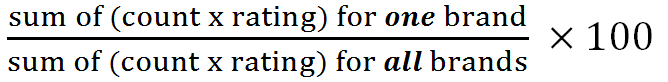This is achieved with the script below: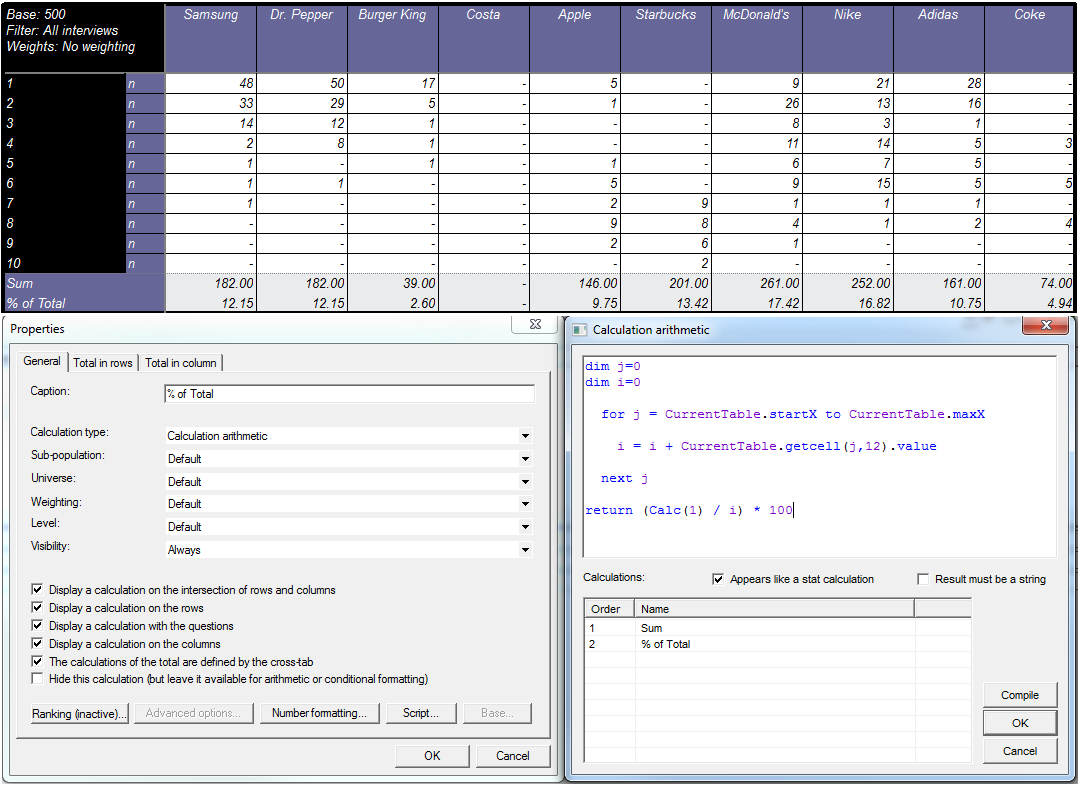A detailed explanation of each line of the script is given in the aforementioned blog post. Here I will show how we achieve this when we add variables into our edge: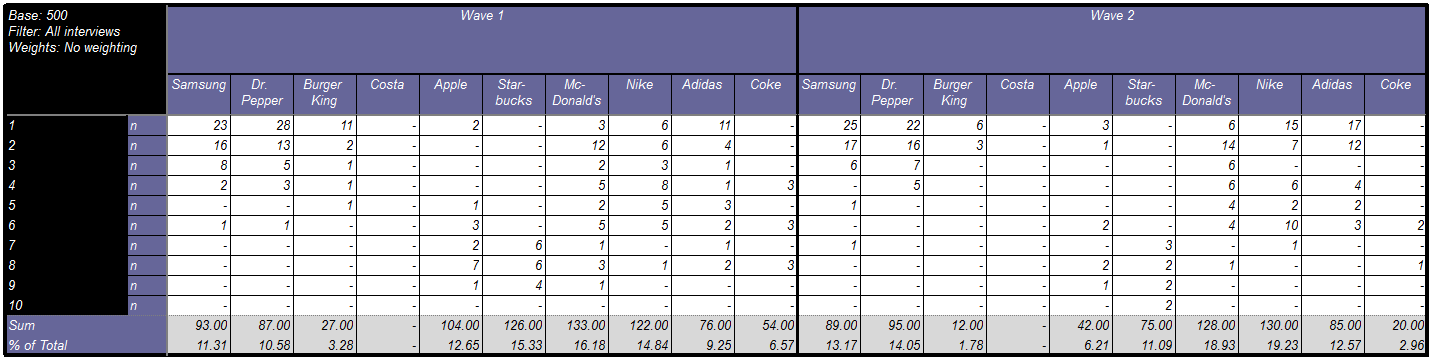This is achieved with the script below: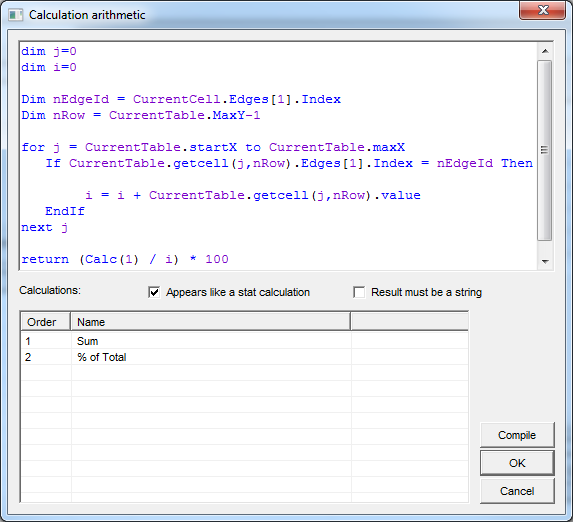Dim nEdgeId = CurrentCell.Edges.Index

This returns the response (order) of the edge we are currently on. So for all columns in the Wave 1 edge it will return 1 and for all columns in the Wave 2 edge it will return 2.

Dim nRow = CurrentTable.MaxY-1

This refers to the second last row in our table (containing figures for the ‘Sum’ calculation).  It’s a dynamic reference.

* In the blog article we used:  i = i + CurrentTable.getcell(j,12).value. However, here we are defining nRow to make this a dynamic reference: i = i + CurrentTable.getcell(j,nRow).value

i.e. if we choose to show another rating in the scale or add another calculation (before ‘Sum’) nRow would automatically account for this, whereas hard coding 12 would mean we’d have to manually update this to 13.

for j = CurrentTable.startX to CurrentTable.maxX

This means the following script will iterate through all columns of the table that contain data (j).

If CurrentTable.getcell(j,nRow).Edges.Index = nEdgeId Then

Firstly we identify what edge the columns belong to. Remember that nEdgeId can take only the values of the number of edge responses you have. In this case it is 1 or 2. Basically the above script is saying do the following summation only for columns in an edge and not across edges.

CurrentTable.getcell(j,nRow).value

EndIf

next j

We sum up all the values contained in these columns (j) for the second to last row (Sum). next j simply means we iterate through each column in turn, incrementing (i) each time until we reach the last column and we have our total sum for all brands (denominator in first formula shown).

Finally:

return (Calc(1) / i) * 100

Calc (1) is the sum of one brand. i is the sum of all brands. We divide the former by the latter to give us the result required from the formula.

The example portfolio contains a tab definition for the example above. There is also a second tab definition which contains slightly amended syntax. The purpose of this is to demonstrate how we would exclude a column from the summation calculation.

In this case we have simply added a calculated response to the variable in the columns for some checks we want to carry out. It shouldn't be included in our bespoke calculation for brands.

We want to end up with this: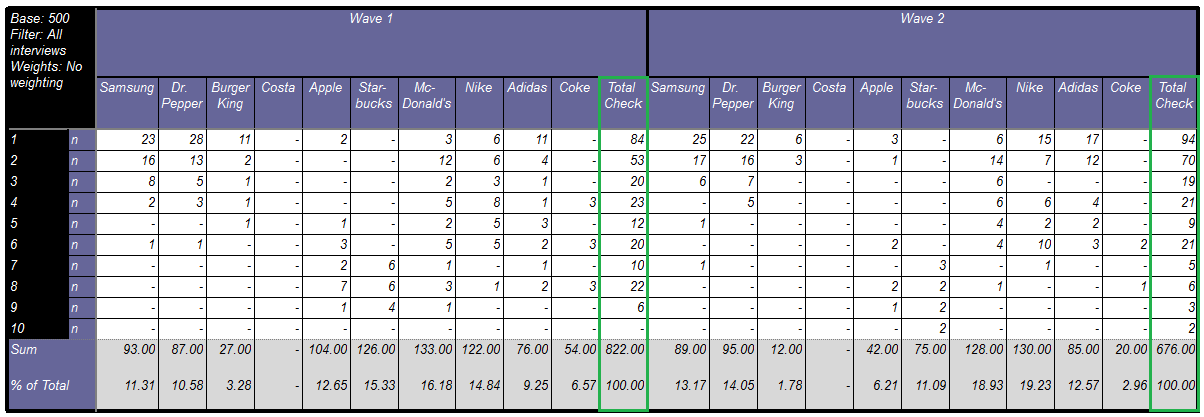The additions to the syntax are explained with the below diagram:

CurrentCell.Column.Question.Responses.Count is picked up from the number of codes available for the first question in your columns.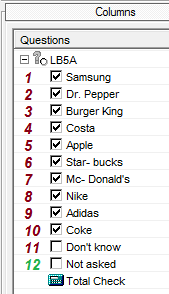Where the syntax to make this work fits in: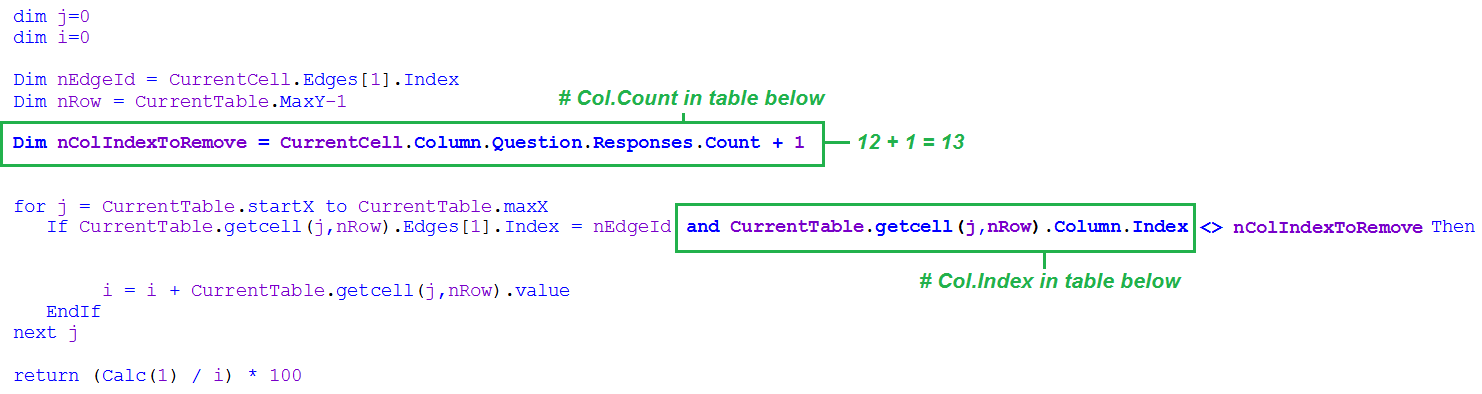Visual representation of what value the syntax takes per column of the table: